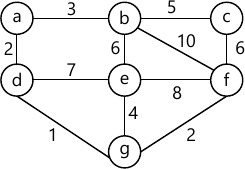MORE IN Data Structures Algorithm
SPPU Computer Engineering (Semester 3)
Data Structures Algorithm
June 2015
Total marks: --
Total time: --
INSTRUCTIONS
(1) Assume appropriate data and state your reasons
(2) Marks are given to the right of every question
(3) Draw neat diagrams wherever necessary

Answer any one question from Q1 and Q2
1 (a) Write a pseudo 'C' code to implement quick sort. Derive time complexity of quick sort in best and worst case.
6 M
1 (b) Derive the code for the following message using Huffman encoding 'A B R A K A D A B R A'.
6 M

2 (a) Sort the following data using merge sort: [10, 5, 15, 3, 20, 1, 30, 9].
3 M
2 (b) Write recursive function to calculate ab.
3 M
2 (c) Create a binary tree from the following inorder and postorder traversals. Also write preorder traversal of the constructed tree:
 Postorder Inorder I D D I H C G G C H F B B F E A A E
3 M
2 (d) What is binary tree ? How is it different from a basic tree ? Explain with figures.
3 M

Answer any one question from Q3 and Q4
3 (a) Write algorithm for Breadth First Traversal of the graph. Also write its complexity.
6 M
3 (b) Construct the AVL tree for the following data: 20, 1, 2, 25, 15, 70, 30, 75, 10, 35. Show clearly rotation used.
6 M

4 (a) Find the shortest path from a to f, in the following graph using Dijkstra's Algorithm.6 M
4 (b) Write 'C' code for the following function w.r.t. AVL tree:
(i) Rotate Left
(ii) Rotate Right
6 M
4 (c) For the hash table size of 10 using hash function key F(key) = key % 10 insert the following keys: 65, 75, 25, 29, 85, 39, 36. Use linear probing with chaining.
3 M

Answer any one question from Q5 and Q6
5 (a) Sort the following data in descending order using heap sort 85, 15, 25, 95, 145, 55, 165, 75. Show all steps.
5 M
5 (b) Construct B+ tree of order 3 for the following data: 10, 2, 30, 5, 90, 100, 50, 75, 35, 25.
4 M
5 (c) Write 'C' program to read 10 integers from keyboard and store them in the file 'My File'.
4 M

6 (a) Create Min Heap for the following data using repeated insertion method 5, 7, 2, 3, 9, 1, 10.
4 M
6 (b) What is B tree ? Explain the procedure to delete node from B tree.
3 M
6 (c) Explain random access file and sequential file.
3 M
6 (d) Explain the following operation on sequential file:
i) Creation
iii) Insert.
3 M

Answer any one question from Q7 and Q8
7 (a) Find the largest number among the following using parallel Computation: 10, 3, 2, 8, 30
6 M
7 (b) Write a parallel algorithm for odd even merge sort.
4 M
7 (c) Explain in detail parallel computation model.
3 M

8 (a) Explain the list ranking problem. Explain with example how will you solve it using pointer jumping techniques.
6 M
8 (b) Compute prefix sum (8, 2, -1, 5) using binary tree techniques.
4 M
8 (c) Write notes on:
i) CRCW
ii) EREW
iii) CREW
3 M

More question papers from Data Structures Algorithm